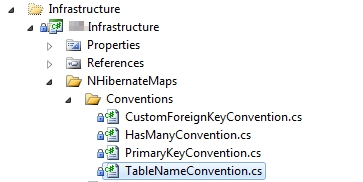# Simple TSQL and C# Entity Generator for S#arp Architecture

By | September 16, 2011

Recently we started a big project which uses S#arp Architecture and for those who does not know what it is you can go to the site (http://www.sharparchitecture.net/) and have a read, but in a gist it is an open source architectural foundation that uses ASP.NET MVC framework with NHibernate.The following principles of this architecture are Domain Driven Design Focus, Loosely coupled, Preconfigured Infrastructure and Open Ended Presentation that means having those principles in mind the developer can concentrate more on Domain and User Experince part of the application that is being developed.

Having said that we used this open-source architecture to leverage on its principles and now we are on the first phase of the project where we are building the Domain layer where we define all the entities needed.  If you have a big database this will be a tedious task mapping tables and columns to a classes so I created a TSQL script to generate those entities so that I’ll just copy and paste the output to a cs file and were ready to go.

```DECLARE @TableName varchar(200)
DECLARE @ProjectName varchar(200) = '<<<YourApplicationName>>>.Domain'
DECLARE @DataType varchar(200)
DECLARE @ColumnName varchar(200)
DECLARE @IsNullable varchar(200)
DECLARE @AppDataType varchar(200) = 'String'
DECLARE @ConstraintType varchar(200)
DECLARE @ReferringTable varchar(200)

DECLARE ClassGenerator CURSOR

--Get all Tables
FOR SELECT TABLE_NAME from <<<YourDatabaseName>>>.INFORMATION_SCHEMA.TABLES where TABLE_TYPE = 'BASE TABLE' ORDER BY TABLE_NAME
OPEN ClassGenerator
FETCH NEXT FROM ClassGenerator
INTO @TableName

PRINT 'namespace ' + @ProjectName
PRINT '{'
PRINT ' using System;'
PRINT ' using SharpArch.Domain.DomainModel;'

WHILE @@FETCH_STATUS = 0
BEGIN

PRINT ' public class ' + dbo.Singularize(@TableName) + ' : Entity'
PRINT ' {'

DECLARE EntityGenerator CURSOR
FOR

--Get all columns and their key types from all the tables
SELECT
COL.COLUMN_NAME,
COL.IS_NULLABLE,
COL.DATA_TYPE,
CST.CONSTRAINT_TYPE,
KCU.TABLE_NAME AS REFERENTIAL_TABLE_SOURCE
FROM
INFORMATION_SCHEMA.KEY_COLUMN_USAGE KCU
INNER JOIN
INFORMATION_SCHEMA.REFERENTIAL_CONSTRAINTS RCN
ON KCU.CONSTRAINT_NAME = RCN.UNIQUE_CONSTRAINT_NAME
RIGHT OUTER JOIN
INFORMATION_SCHEMA.KEY_COLUMN_USAGE KCU2
INNER JOIN
INFORMATION_SCHEMA.TABLE_CONSTRAINTS CST
ON KCU2.CONSTRAINT_NAME = CST.CONSTRAINT_NAME
AND KCU2.TABLE_NAME = CST.TABLE_NAME
ON RCN.CONSTRAINT_NAME = KCU2.CONSTRAINT_NAME
RIGHT OUTER JOIN
INFORMATION_SCHEMA.COLUMNS COL
ON KCU2.TABLE_NAME = COL.TABLE_NAME
AND KCU2.COLUMN_NAME = COL.COLUMN_NAME
WHERE
COL.TABLE_NAME = @TableName
ORDER BY
COL.TABLE_NAME

OPEN EntityGenerator
FETCH NEXT FROM EntityGenerator
INTO @ColumnName, @IsNullable, @DataType, @ConstraintType, @ReferringTable

WHILE @@FETCH_STATUS = 0
BEGIN

IF @ConstraintType <> 'PRIMARY KEY' OR @ConstraintType IS NULL
BEGIN
IF @ConstraintType = 'FOREIGN KEY'
BEGIN
PRINT ' public virtual ' + dbo.Singularize(@ReferringTable) + ' '  + dbo.Singularize(@ReferringTable) + ' { get; set; }'
END
ELSE
BEGIN

--SQL to .NET Data Type Mapping
IF @DataType = 'bigint' BEGIN SET @AppDataType = 'Int64' END
IF @DataType = 'binary' BEGIN SET @AppDataType = 'Byte[]' END
IF @DataType = 'bit' BEGIN SET @AppDataType = 'Boolean' END
IF @DataType = 'char' BEGIN SET @AppDataType = 'String' END
IF @DataType = 'date' BEGIN SET @AppDataType = 'DateTime' END
IF @DataType = 'datetime' BEGIN SET @AppDataType = 'DateTime' END
IF @DataType = 'datetimeoffset' BEGIN SET @AppDataType = 'DateTimeOffset' END
IF @DataType = 'decimal' BEGIN SET @AppDataType = 'decimal' END
IF @DataType = 'float' BEGIN SET @AppDataType = 'Double' END
IF @DataType = 'image' BEGIN SET @AppDataType = 'Byte[]' END
IF @DataType = 'int' BEGIN SET @AppDataType = 'Int32' END
IF @DataType = 'money' BEGIN SET @AppDataType = 'Decimal' END
IF @DataType = 'nchar' BEGIN SET @AppDataType = 'String' END
IF @DataType = 'ntext' BEGIN SET @AppDataType = 'String' END
IF @DataType = 'numeric' BEGIN SET @AppDataType = 'Decimal' END
IF @DataType = 'nvarchar' BEGIN SET @AppDataType = 'String' END
IF @DataType = 'real' BEGIN SET @AppDataType = 'Single' END
IF @DataType = 'rowversion' BEGIN SET @AppDataType = 'Byte[]' END
IF @DataType = 'smalldatetime' BEGIN SET @AppDataType = 'DateTime' END
IF @DataType = 'smallint' BEGIN SET @AppDataType = 'Int16' END
IF @DataType = 'smallmoney' BEGIN SET @AppDataType = 'Decimal' END
IF @DataType = 'sql_variant' BEGIN SET @AppDataType = 'Object' END
IF @DataType = 'text' BEGIN SET @AppDataType = 'String' END
IF @DataType = 'time' BEGIN SET @AppDataType = 'TimeSpan' END
IF @DataType = 'timestamp' BEGIN SET @AppDataType = 'Byte[]' END
IF @DataType = 'tinyint' BEGIN SET @AppDataType = 'Byte' END
IF @DataType = 'uniqueidentifier' BEGIN SET @AppDataType = 'Guid' END
IF @DataType = 'varbinary' BEGIN SET @AppDataType = 'Byte[]' END
IF @DataType = 'varchar' BEGIN SET @AppDataType = 'String' END
IF @DataType = 'xml' BEGIN SET @AppDataType = 'Xml' END

IF @IsNullable = 'YES' AND @AppDataType <> 'Byte[]' AND @AppDataType <> 'String'
BEGIN
PRINT ' public virtual ' + @AppDataType + '? ' + @ColumnName + ' { get; set; }'
END
ELSE
BEGIN
PRINT ' public virtual ' + @AppDataType + ' ' + @ColumnName + ' { get; set; }'
END
END
END
FETCH NEXT FROM EntityGenerator
INTO @ColumnName, @IsNullable, @DataType, @ConstraintType, @ReferringTable

END
CLOSE EntityGenerator
DEALLOCATE EntityGenerator

PRINT ' }'

FETCH NEXT FROM ClassGenerator

INTO @TableName
END
PRINT '}'
CLOSE ClassGenerator
DEALLOCATE ClassGenerator```

Now I noticed wouldn’t it be cleaner to create each entity in different class files? So I created a console application to do that which separates each entity to a file.  And here is the code:

```using System;
using System.Collections.Generic;
using System.Linq;
using System.Text;
using System.Data;
using System.Data.SqlClient;
using System.IO;

class Program
{
static void Main(string[] args)
{
string sConnString = "Data Source=<<<YourDatabaseServer>>>;Initial Catalog=<<<YourDatabase>>;Integrated Security=SSPI";
SqlConnection oSQLConn1 = new SqlConnection(sConnString);
SqlDataReader oReader = null;
string sProjectName = "<<<YourProjectName>>>.Domain";
string sGeneratedCodeLocation = @"C:<<<YourProjectFolder>>>";

oSQLConn1.Open();

SqlCommand oCommand = new SqlCommand("SELECT TABLE_NAME, dbo.Singularize(TABLE_NAME) as SINGULARIZED_TABLE from INFORMATION_SCHEMA.TABLES WHERE TABLE_TYPE = 'BASE TABLE' ORDER BY TABLE_NAME", oSQLConn1);

oReader = oCommand.ExecuteReader();

while (oReader.Read())
{
string sTableName = oReader.ToString();
string sSingularizedTableName = oReader.ToString();
SqlConnection oSQLConn2 = new SqlConnection(sConnString);
SqlDataReader oItemReader = null;

oSQLConn2.Open();

SqlCommand oItemCommand =
new SqlCommand(@"SELECT
COL.COLUMN_NAME,
COL.IS_NULLABLE,
COL.DATA_TYPE,
CST.CONSTRAINT_TYPE,
dbo.Singularize(KCU.TABLE_NAME) AS REFERENTIAL_TABLE_SOURCE
FROM
INFORMATION_SCHEMA.KEY_COLUMN_USAGE KCU
INNER JOIN
INFORMATION_SCHEMA.REFERENTIAL_CONSTRAINTS RCN
ON KCU.CONSTRAINT_NAME = RCN.UNIQUE_CONSTRAINT_NAME
RIGHT OUTER JOIN
INFORMATION_SCHEMA.KEY_COLUMN_USAGE KCU2
INNER JOIN
INFORMATION_SCHEMA.TABLE_CONSTRAINTS CST
ON KCU2.CONSTRAINT_NAME = CST.CONSTRAINT_NAME
AND KCU2.TABLE_NAME = CST.TABLE_NAME
ON RCN.CONSTRAINT_NAME = KCU2.CONSTRAINT_NAME
RIGHT OUTER JOIN
INFORMATION_SCHEMA.COLUMNS COL
ON KCU2.TABLE_NAME = COL.TABLE_NAME
AND KCU2.COLUMN_NAME = COL.COLUMN_NAME
WHERE
COL.TABLE_NAME = '" + sTableName +
@"' ORDER BY
COL.TABLE_NAME", oSQLConn2);

oItemReader = oItemCommand.ExecuteReader();
TextWriter oCSFile = new StreamWriter(sGeneratedCodeLocation + sSingularizedTableName + ".cs");

oCSFile.WriteLine("namespace " + sProjectName);
oCSFile.WriteLine("{");
oCSFile.WriteLine("tusing System;");
oCSFile.WriteLine("tusing SharpArch.Domain.DomainModel;");

oCSFile.WriteLine("tpublic class " + sSingularizedTableName + " : Entity");
oCSFile.WriteLine("t{");

while (oItemReader.Read())
{
string sAppDataType = "String";

string sColumnName = oItemReader.ToString();
string sIsNullable = oItemReader.ToString();
string sDataType = oItemReader.ToString();
string sConstraintType = oItemReader.ToString();
string sReferringTable = oItemReader.ToString();

if (sConstraintType != "PRIMARY KEY" || sConstraintType == null)
{
if (sConstraintType == "FOREIGN KEY")
{
oCSFile.WriteLine("ttpublic virtual " + sReferringTable + " " + sReferringTable + " { get; set; }");
}
else
{
//SQL to .NET Data Type Mapping
if (sDataType == "bigint") sAppDataType = "Int64";
if (sDataType == "binary") sAppDataType = "Byte[]";
if (sDataType == "bit") sAppDataType = "Boolean";
if (sDataType == "char") sAppDataType = "String";
if (sDataType == "date") sAppDataType = "DateTime";
if (sDataType == "datetime") sAppDataType = "DateTime";
if (sDataType == "datetimeoffset") sAppDataType = "DateTimeOffset";
if (sDataType == "decimal") sAppDataType = "decimal";
if (sDataType == "float") sAppDataType = "Double";
if (sDataType == "image") sAppDataType = "Byte[]";
if (sDataType == "int") sAppDataType = "Int32";
if (sDataType == "money") sAppDataType = "Decimal";
if (sDataType == "nchar") sAppDataType = "String";
if (sDataType == "ntext") sAppDataType = "String";
if (sDataType == "numeric") sAppDataType = "Decimal";
if (sDataType == "nvarchar") sAppDataType = "String";
if (sDataType == "real") sAppDataType = "Single";
if (sDataType == "rowversion") sAppDataType = "Byte[]";
if (sDataType == "smalldatetime") sAppDataType = "DateTime";
if (sDataType == "smallint") sAppDataType = "Int16";
if (sDataType == "smallmoney") sAppDataType = "Decimal";
if (sDataType == "sql_variant") sAppDataType = "Object";
if (sDataType == "text") sAppDataType = "String";
if (sDataType == "time") sAppDataType = "TimeSpan";
if (sDataType == "timestamp") sAppDataType = "Byte[]";
if (sDataType == "tinyint") sAppDataType = "Byte";
if (sDataType == "uniqueidentifier") sAppDataType = "Guid";
if (sDataType == "varbinary") sAppDataType = "Byte[]";
if (sDataType == "varchar") sAppDataType = "String";
if (sDataType == "xml") sAppDataType = "Xml";

if (sIsNullable == "YES" && sAppDataType != "Byte[]" && sAppDataType != "String")
{
oCSFile.WriteLine("ttpublic virtual " + sAppDataType + "? " + sColumnName + " { get; set; }");
}
else
{
oCSFile.WriteLine("ttpublic virtual " + sAppDataType + " " + sColumnName + " { get; set; }");
}
}

}
}
oCSFile.WriteLine("t}");
if (oItemReader != null) oItemReader.Close();
if (oSQLConn2 != null) oSQLConn2.Close();

oCSFile.WriteLine("}");
oCSFile.Close();
}
Console.ReadLine();

if (oReader != null) oReader.Close();
if (oSQLConn1 != null) oSQLConn1.Close();
}
}```

Now lets dissect the codes, if you noticed I used a Singularize Function to Singularize Table Names I have posted a code for that which you can find here
http://www.macaalay.com/2011/09/13/singularize-function-in-tsql/
.

Now let’s go to the queries, you will notice that there is a complex join in how we get our data regarding our data structure which can be easily extracted from the Information Schema, if you are interested on what other items can be extracted from it have a read here
http://msdn.microsoft.com/en-us/library/ms186778.aspx

Once we have the data we need, we start to process it and it’s not that straightforward but its easy all you need to do is to gather information whether a column or field is nullable so that we can declare it as nullabe in the codes.  We also need a mapping to SQL DataTypes to .Net Data Types hence the big if conditions.

Also since we are using the S#arp Architecture there is a convention for PrimaryKeys so we don’t need to declare it as it is handled by the PrimaryKeyConvention class under NHibernateMapsConvention, so if you named your PK right prefixing them with Id then this will be easy but you can always override it if you wanted to.  Below is the code that handles it```namespace CI5.Infrastructure.NHibernateMaps.Conventions
{
#region Using Directives

using FluentNHibernate.Conventions;

#endregion

public class PrimaryKeyConvention : IIdConvention
{
public void Apply(FluentNHibernate.Conventions.Instances.IIdentityInstance instance)
{
instance.Column(instance.EntityType.Name + "Id");
}
}
}```

Finally we need to take note of Foreign Keys and what table is it related to as we don’t declare Id’s in the Entities but the class it is related to, hence we have this line.

```if (sConstraintType == "FOREIGN KEY")
{
oCSFile.WriteLine("ttpublic virtual " + sReferringTable + " " + sReferringTable + " { get; set; }");
}```

Other than that everything should be straightforward.

The above code is made to generate only basic stuff so if you have added validation and other lines in your entities do not use it, otherwise I hope this would make someones life easier.

###### Recommended

This site uses Akismet to reduce spam. Learn how your comment data is processed.